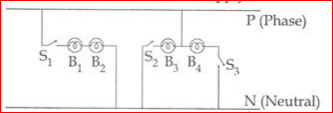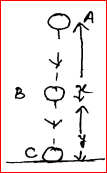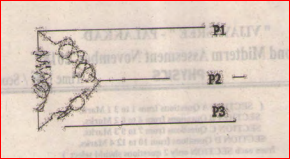# Kerala SSLC Class 10 Physics Important Questions

Learn important points from the chapters of Kerala Board SSLC Physics with the help of some important questions which we at BYJU’s have compiled following an analysis of the Kerala SSLC previous year question papers. This attracts the students to the subject and they also get an insight into the type of questions normally asked for Kerala SSLC exams.

Some of the questions normally found on the major topics as per the Kerala sslc syllabus are on effects of electric current, wave motion, heat, colours of light and so on. Students who solve these Kerala SSLC Class 10 Physics Important Questions are also better equipped to answer the board exams without much fear.

How about an overview of the list of important questions we have compiled for Class 10 Physics Kerala board?

1. Roshan observed a beautiful rainbow in the western SKY from his school ground.

(b) When did he observe the rainbow?

[Morning, noon, evening, prediction of time impossible]

(b)Draw the diagram of dispersion taking place in a water droplet during the formation of a rainbow

2. (a) Write one example each for fossil fuels in solid and liquid state.

(b) Which fossil fuel produces Ammonia when distilled in the absence of air

(c)Write down the process of evolution of fossil fuels on earth

3. Four [40W, 2300V] bulbs are connected to a 230 V supply as shown in the diagram below:1. Which are the bulbs connected in the series
2. Which are the bulbs that can work at 40 W power
3. Write two advantages of connecting household equipment in parallel

4. Name the material used for (i) heating element of a room heater, (ii) fuse wire. Give reasons for your answer. ‘

5. Two bodies of masses m and 2m are placed at a height h. Find the ratio of their gravitational potential energy.

6. A ray of light is normally incident on one face of an equilateral glass prism. Answer the following :

(a) What is the angle of incidence on the first face of the prism ?

(b) What is the angle of refraction from the first face of the prism?

7. Derive the relationship between SI and CGS unit of work.

8. An iron door of a building is 3m broad. It can be opened by applying a force of 100 N normally at the middle of the door. Calculate (a) the torque needed to open the door, (b) the least force and its point of application to open the door.

9. An object of mass m is allowed to fall freely from point A as shown in the figure below. Calculate the total mechanical ene10. Dispersion of light through water droplets in the atmosphere causes rainbow.

1. Draw a sketch of dispersion of light through water droplet
2. The rainbow is seen as a circle when viewed from an aeroplane flying at high altitudes. Give reason?

11. A transformer without power loss has 500turns in its primary coil and 2500 turns in the secondary coil. It induces a potential difference of 250 V and 0.2 A in the secondary.

1. Name the type of transformer
2. Find the voltage in the primary coil
3. What is the intensity of current in the primary coil

12. Why does the earth pin differ from the other pins in a 3-pin plug?

13. Star connection is wrongly depicted in the picture given below:1. Redraw the correct figure.
2. What is the potential difference between the neutral line and the earth?

14. L.P.G is commonly used as a domestic fuel.

1. What are its main constituents?
2. Write the expanded form of L.P.G.
3. L.P.G is a colorless, odourless gas.However, L.P.G used for domestic purposes has an odour. What is the reason?

15. Spirit taken in a watch glass disappears into air without even heating.

1. Write the name of the above phenomenon
2. Explain a daily life situation where the above phenomenon is used